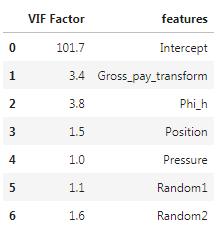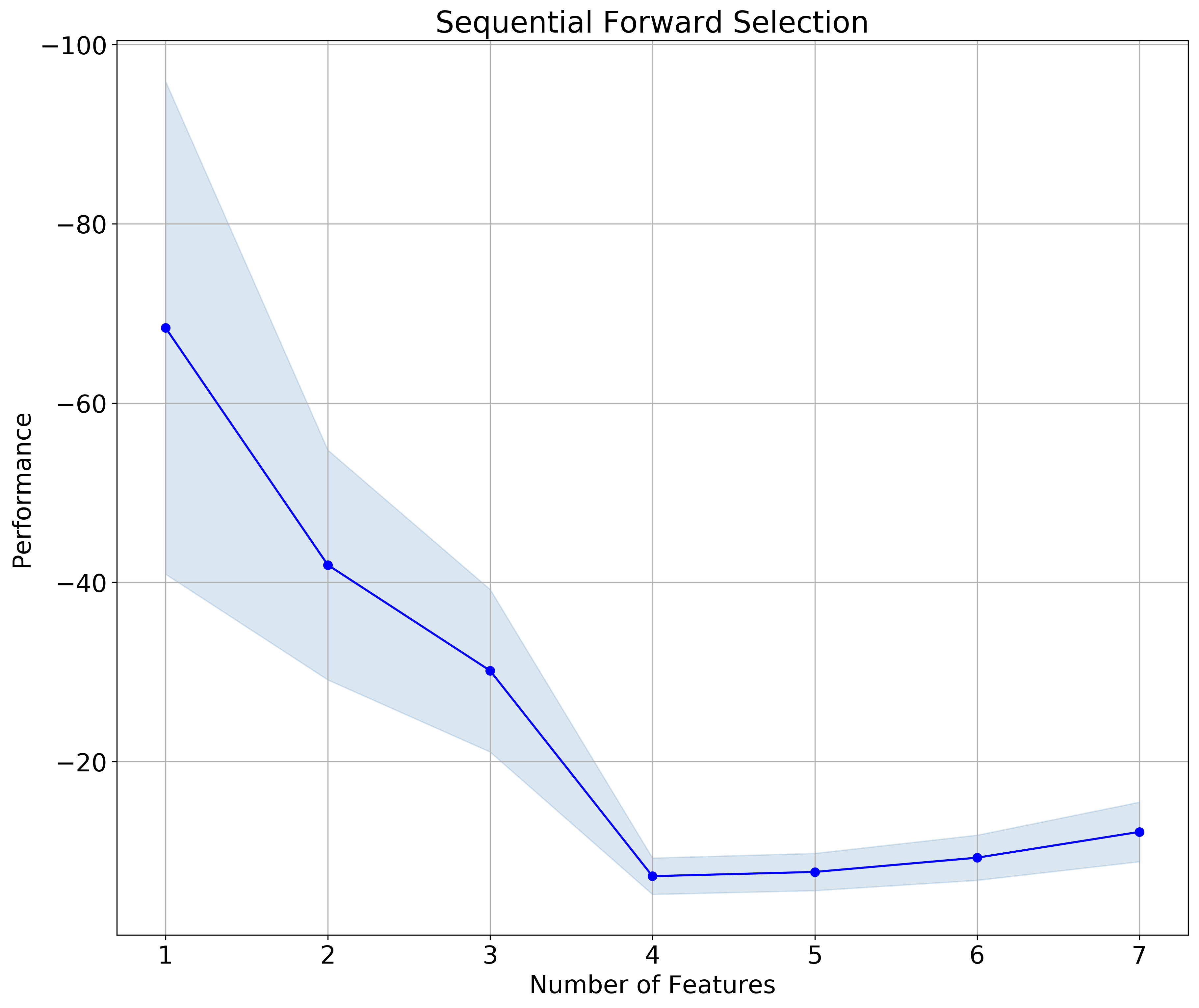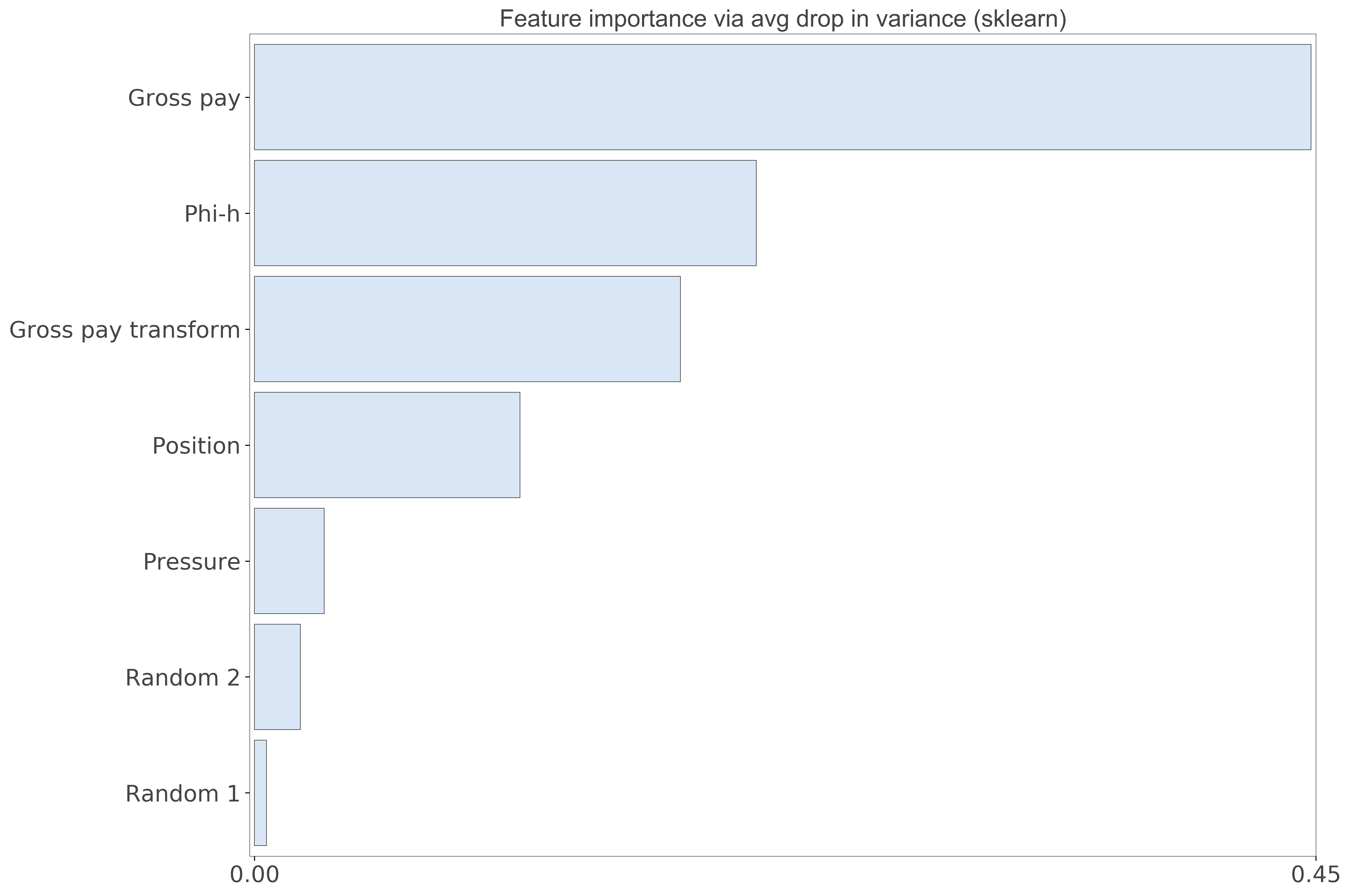# Variable selection in Python, part I

#### Introduction and recap

In my previous two posts of this (now official, but) informal Data Science series I worked through some strategies for doing visual data exploration in Python, assisted by domain knowledge and inferential tests (rank correlation, confidence, spuriousness), and then extended the discussion to more robust approaches involving distance correlation and variable clustering.

For those that have not read those posts, I am using a dataset comprising 21 wells producing oil from a marine barrier sand reservoir; the data was first published by Lee Hunt in 2013 in a CSEG Recorder paper titled Many correlation coefficients, null hypotheses, and high value.

Oil production, the dependent variable, is measured in tens of barrels of oil per day (it’s a rate, actually). The independent variables are: Gross Pay, in meters; Phi-h, porosity multiplied by thickness, with a 3% porosity cut-off; Position within the reservoir (a ranked variable, with 1.0 representing the uppermost geological facies, 2.0 the middle one, 3.0 the lowest one); Pressure draw-down in MPa. Three additional ‘special’ variables are: Random 1 and Random 2, which are range bound and random, and were included in the paper, and Gross Pay Transform, which I created specifically for this exercise to be highly correlated to Gross pay, by passing Gross pay to a logarithmic function, and then adding a bit of normally distributed random noise.

#### Next step: variable selection (Jupyter Notebooks here)

The idea of variable selection is to try to understand which independent variables are more and which are less important in predicting the dependent variable (Production in this case), and also which ones may be highly correlated to one another (in other words, they carrying the same information); in both cases, assisted by domain knowledge, we drop some of the variables, resulting (ideally) in an improved prediction by a model that is simpler and can generalize better.

I really love the systematic way in which Thomas, working on the same dataset but using R, looked at several methods for variable selection and then summarized all the results in a table. The insight from this (quite) exhaustive analysis helped him chose a subset of variables to use in the final regression. I really, REALLY recommend reading his interactive R notebook.

As for me, one of the goals I had in mind at the end of our 2018 collaboration on this project was to be able to do something similar in Python, and I am delighted to say I think I was able to achieve that goal.

In this post I will look at:

• Distance correlation, again
• Multicollinearity, using Variance Inflation Factor (VIF)
• Sequential feature selection, using both a backward and forward approach
• Random Forest Regressor variable importance, using a drop-column approach
• Multicollinearity, using variable dependence

In the next (1 or 2) post(s) I will look at:

• Permutation importance using an Extra Tree Regressor
• Mutual information
• The relative magnitude of the transformed variables in ACE (Alternating Conditional Expectation)
• SHAP values (Shapley additive explanations)
• The sign of the weights of a neural network (excitory (positive weights) vs. inhibitory (negative weighs))

I think this is a good mix as it combines methods and then summarize the results from all methods.

##### Distance correlation

in Figure 1, below, I plot again the correlation matrix of bivariate scatterplots, rearranged according to the clustering results from last post, and with the distance correlation annotated and coloured by its bootstrapping p-value.

Phi-h, Gross Pay, and Gross pay transform are highly correlation to Production, with statistical significance at the 10%level given by the p-value. However, there is a good chance also also of multicollinearity at play, almost certainly between Gross Pay and Gross Pay Transform, with a DC of 0.97; we know why, in this case, imposed it in this case, but we might have not known.Figure 1. Seaborn pairgrid matrix with distance correlation colored by p-value (gray if > 0.10, blue if p <= 0.10), and plots rearranged by clustering results

##### Variance Inflation Factor (VIF)

Variance inflation factor (VIF) is a technique to estimate the severity of multicollinearity among independent variables within the context of a regression. It is calculated as the ratio of all the variances in a model with multiple terms, divided by the variance of a model with one term alone.

First, we set-up a regression, using the Patsy library to generate design matrices for target and predictors:

```outcome, predictors = dmatrices("Production ~ Gross_pay +Phi_h +Position
+Pressure +Random1 +Random2 +Gross_pay_transform",
data, return_type='dataframe')
```

for which then VIF factors can be calculated with:

```vif["VIF Factor"] = [variance_inflation_factor(predictors.values, i)
for i in range(predictors.shape)]```

The values are summarized in Table I below; variables that have variance inflation factor that is high (ignoring the intercept) and similar in value have a high chance of being collinear because they explain the same variance in the dataset.Table I. Regression VIF factors

For this model, the result suggests either Gross Pay or Gross Pay Transform should be dropped, otherwise the risk is of building a model with high multicollinearity (that is, predictions would be very susceptible to small noise fluctuations).

But which one should we drop? It occurred to me that one possibility would be to drop one in turn and recalculate the VIF factors.Table II. VIF after dropping Gross Pay Transform

As seen in Table II, after removing Gross Pay Transform  all VIF factors are below the cut-off value of 5 (rule-of-thumb suggested in this article, and reference therein). I  would make the additional observation. that because the factors for Phi-h and Gross Pay are now close, even though below the cutoff,  there may be some (smaller amount of) collinearity between the two variables, which is consistent to be expected since both variables contain some information on height (one of pay, one of porosity).

We see something similar when removing Gross Pay; in fact, the Factors for Gross Pay Transform and Phi-h in Table III are also close, yes, but smaller. I’d conclude that VIF is veru sueful in highlighting multicollinearity, but it does not necessarily answer the question of which collinear feature shoud be dropped.Table III. VIF after dropping Gross Pay

##### Sequential feature selection

Sequential feature selection (similarly to Scikit-learn’s Recursive Feature Elimination) is used  “to automatically select a subset of features that is most relevant to the problem. The goal of feature selection is two-fold: we want to improve the computational efficiency and reduce the generalization error of the model by removing irrelevant features or noise”.

I tested both Sequential Forward Selection (SFS) and Sequential Backward Selection (SBS) from Sebastan Raschka‘s  `mlxtend` library to search for that optimal subset of features (for a full overview of the method, and a great set of detailed examples, please see the excellent documentation by Sebastian). You can download and run the full notebook fro the GitHub repo here).

The only difference between SFFS and SBFS is that the former starts with at 1 feature and adds them one by one, whereas the latter starts with all features (or a user defined pre-selected number) and removes them one by one. In both cases I used the selector as part of a pipeline including Scikit-learn’s linear regression and cross-validation with Leave One Out (i.e., dropping one well at a time); for example, the pipeline for SFS is:

```features = data.loc[:, ['Position', 'Gross pay', 'Phi-h', 'Pressure',
'Random 1', 'Random 2', 'Gross pay transform']].values
y = data.loc[:, ['Production']].values

LR = LinearRegression()
loo = LeaveOneOut()

sfs = SFS(estimator=LR,
k_features=7,
forward=True,
floating=True,
scoring='neg_mean_squared_error',
cv = loo,
n_jobs = -1)
sfs = sfs.fit(X, y)
```

and the feature selection results are plotted in Figure 2, generated with a modified version of Sebastian’s `mlxtend` utility function:

```plot_sequential_feature_selection(sfs.get_metric_dict(), kind='std_err')
plt.gca().invert_yaxis()
```

Please notice that having flipped the y axis (my personal preference), performance for SFFS (as given by negative mean square error) improves towards the bottom.Figure 2. Sequential Forward Selection

The results for SFBS is plotted in Figure 3. Notice that in this case I flipped both the y axis  and the x axis; the latter makes the sequential selection go from left to right, which I find a bit more intuitive, given we read from left to right.Figure 3. Sequential Backward Selection

In both cases the subset is made up of 4 feature, and – to my delight !! –  the selected features are the same (check the notebook to see how I extract the information):

```>>>  ['Position', 'Gross pay', 'Phi-h', 'Pressure']
```
##### Drop-column feature importance

You can download the notebook for both drop-column importance and dependence from here.

I have to say I’ve never been comfortable with using Feature Importance plots you get from Random Forest. In part because, on occasion, I noticed a disconnect with what domain knowledge-informed intuition would suggest; in part, I confess, because I thought (and I was right) I had an incomplete understanding of what goes on in the background. Until I read the article How to not use random forest. The example with toy dataset in there is not the most exciting, but it demonstrate clearly how using Feature Importance with preset parameters places a random variable at the top. If you wonder how can that be, I recommend reading the article.

Or read on, there’s more coming: curious, I did some more searching, and found this article, Selecting good features – Part III: random forests. There’s a nicer example in there, using the Boston Housing dataset, and to me a clearer explanation of why one should not use the default Scikit-learn Mean Decrease Impurity metric (strong, but correlated features can end up with low scores).

Finally, I found Beware Default Random Forest Importances, where the authors (thank you!!!) not only walk readers through a full set of experiments, run in both Python and R, but provide a great library (called `rfpimp`), to do your own work in Python.

I really like their drop-column importance, which is implemented to answers the question of how important a feature is to the overall model performance … and does it  … even more directly than the permutation importance.

That is achieved with a brute force drop-column apprach involving:

• training the model with all features to get a baseline performance score
• dropping a column
• retraining  the model and recomputing the performance score.

The importance value of a feature is then the difference between the baseline and the score from the model without that feature.

I also REALLY like that unimportant features do not have just very low importance; some do, but some have negative importance, exposing that removing them improves model performance. This is the case, with our small dataset of the Random 1 and Random 2 variables, as shown in Figure 4. It is also the case of Pressure. Of the remaining variables, Gross Pay Transform has very low importance (please notice the range is 0-0.15 for this plot, a conscious choice by the authors), Gross pay and Phi-h look somewhat important, and Position in the reservoir is the most important feature. This is excellent insight; please compare to the importances with Scikit-learn’s defautl metric, in Figure 5.Figure 4. rfpimp Drop-column importance. Notice the 0-0.15 rangeFigure 5. Scikit-learn Feature importance. Notice the 0-0.45 range

##### Dependence

This last analysis is similar to Thomas’ Redundancy Analysis in that we look for those variables that can be predicted using the other variables.  Using the `feature_dependence_matrix` function from the `rfpimp` library we get:

```>>> Dependence:
Gross pay               0.939
Gross pay transform     0.815
Phi-h                   0.503
Random 2               0.0789
Position               0.0745
Pressure               -0.396
Random 1               -0.836

```

By removing Gross Pay Transform, and repeating the analysis, we get:

```>>> Dependence:
Gross pay    0.594
Phi-h        0.573
Random 2     0.179
Position     0.106
Pressure    -0.339
Random 1    -0.767```

and by removing Gross Pay:

```>>> Dependence:
Gross pay transform     0.479
Phi-h                   0.429
Position                0.146
Random 2              -0.0522
Pressure               -0.319
Random 1               -0.457

```

These results show, again, that either Gross Pay or Gross Pay Transform should be dropped (perhaps the former), because of very high chance of dependence (~multicollinearity). Also Phi-h is somewhat predictable from the other variables, but not as much, so it may be fine, if not good, to keep it (that’s what domain knowledge would suggest).

They are in agreement with the results from VIF, but this time the outcome is blind to the outcome (the target Production) so I’d consider it more robust.